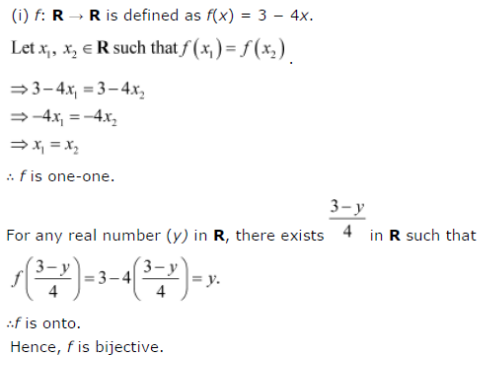# In each of the following cases, state whether the function is one-one,

In each of the following cases, state whether the function is one-one, onto or bijective. Justify your answer.
(i) f : R → R defined by f(x) = 3 - 4x
(ii) f : R → R defined by f(x) = 1 + x2